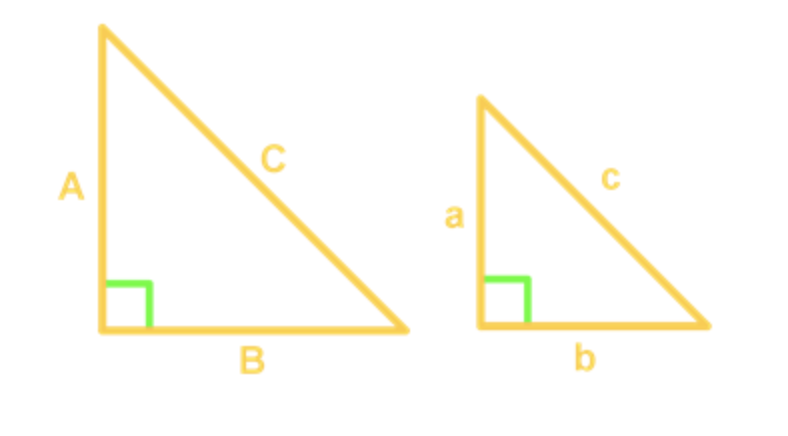# Converting among ratios, fractions and decimals#### Everything You Need in One Place

Homework problems? Exam preparation? Trying to grasp a concept or just brushing up the basics? Our extensive help & practice library have got you covered.#### Learn and Practice With Ease

Our proven video lessons ease you through problems quickly, and you get tonnes of friendly practice on questions that trip students up on tests and finals.#### Instant and Unlimited Help

Our personalized learning platform enables you to instantly find the exact walkthrough to your specific type of question. Activate unlimited help now!

##### Intros
###### Lessons
1. Introduction to Converting Among Ratios, Fractions and Decimals
##### Examples
###### Lessons
1. Converting Ratios to Fractional and Decimal Forms

A questionnaire shows that the ratio of iPhone to Samsung users is 4:5.

1. Express the ratio in fractional form.
2. Convert the ratio to its decimal form.
2. Part-to-part Vs. Part-to-whole Ratios

Dennis drew a picture using only 14 circles and 12 triangles.

1. What is the ratio of circles to triangles in fractional form?
2. What is the ratio of circles to all the shapes in the picture in fractional form?
3. Use of Fractional Ratios

The ratio of males to females in a certain class is 2 to 3. How many of the 45 students are female?

1. Comparing Ratios

Find the value of x for the following ratios.

1. $x:12 = 1:2$
2. $\frac{(2x+3)}{4} = \frac{(x+1)}{2}$
2. Application of Comparing Ratios

The ratio of horses and cows at a farm is 10:13. If there are 91 cows, how many horses are there?

1. Application of Ratios - Tips

The tips Mary paid on her $800 meal is$120. How much would she have to pay for tips if a person had a meal of \$240?

1. Application of Ratios – Similar Triangles

Given the following similar triangles. If A=32, B=24, C=40 and b=3, find the lengths of sides a and c.###### Topic Notes
In this lesson, we will learn:
• Converting Ratios to Fractional and Decimal Forms
• Part-to-part Vs. Part-to-whole Ratios
• Use of Fractional Ratios
• Comparing Ratios
• Application of Comparing Ratios
• Application of Ratios – Tips
• Application of Ratios – Similar Triangles

Notes:
• A ratio in the form of $a:b$ can be written in fractional form $\frac{a}{b}$, which can be further converted to decimals by solving a÷b.